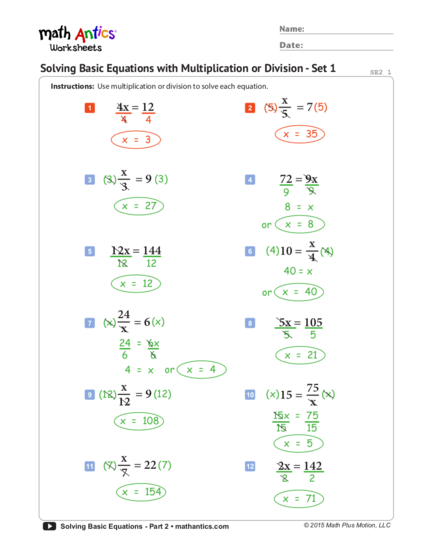# Solving Basic Equations with Multiplication or DivisionContributed by:Solving Basic Equations with Multiplication or Division question and answers. Sample questions along with answers for a better understanding of the topic.
1. ® Name:
Date:
Solving Basic Equations with Multiplication or Division - Set 1 SE2 1
Instructions: Use multiplication or division to solve each equation.
x
1 4x = 12 2 (5) = 7 (5)
4 4
5
x = 35
x = 3
x
3 (3) = 9 (3) 4 72 = 9x
3 9 9
x = 27 8 = x
or x = 8
x
5 12x = 144 6 (4) 10 = (4)
12 12
4
40 = x
x = 12
or x = 40
24
7 (x) = 6 (x) 8 5x = 105
x 5 5
24 = 6x
6 6 x = 21
4 = x or x = 4
x 75 (x)
9 (12) = 9 (12) 10 (x) 15 =
12 x
15x = 75
x = 108
15 15
x = 5
x
11 (7) = 22 (7) 12 2x = 142
7 2 2
x = 154
x = 71
Solving Basic Equations - Part 2 • mathantics.com © 2015 Math Plus Motion, LLC
2. ® Name:
Date:
Solving Basic Equations with Multiplication or Division - Set 2 SE2 2
Instructions: Use multiplication or division to solve each equation.
48 (x)
1 40 = 8x 2 (x) 12 =
8 8 x
12x = 48
5 = x
12 12
or x = 5 x = 4
x
3 (8) = 8 (8) 4 11x = 66
8 11 11
x = 64
x = 6
32 x
5 (x) = 4 (x) 6 (3) = 24 (3)
x 3
32 = 4x
x = 72
4 4
8 = x or x = 8
x
7 6x = 78 8 (4) = 14 (4)
6 6 4
x = 56
x = 13
84 (x)
9 (x) 7 = 10 65 = 5x
x 5 5
7x = 84
13 = x
7 7
x = 12 or x = 13
x
11 3x = 135 12 (20) 3 = (20)
3 3
20
60 = x
x = 45
or x = 60
Solving Basic Equations - Part 2 • mathantics.com © 2015 Math Plus Motion, LLC
3. ® Name:
Date:
Solving Basic Equations (with Decimals) SE2 3
Instructions: Use multiplication or division to solve each equation. You can use a calculator to do
the decimal arithmetic if you’d like to.
x
1 5.0 = 2.5x 2 (2) = 1.6 (2)
2.5 2.5 2
2 = x x = 3.2
or x = 2
3 (x)1.5 =
0.5 (x) 4 0.1x = 2.4
x 0.1 0.1
1.5x = 0.5
1.5 1.5 x = 24
x = 0.3
x 3.5
5 (2.1) = 1.6 (2.1) 6 (x) = 2.5 (x)
2.1 x
x = 3.36 3.5 = 2.5x
2.5 2.5
1.4 = x or x = 1.4
x
7 (3) = 6.4 (3) 8 0.2x = 0.7
3 0.2 0.2
x = 19.2
x = 3.5
8.4 (x)
9 (x) 8 = 10 2.25 = 0.75x
x 0.75 0.75
8x = 8.4
3 = x
8 8
x = 1.05 or x = 3
Solving Basic Equations - Part 2 • mathantics.com © 2015 Math Plus Motion, LLC
4. ® Name:
Date:
Solving Basic Equations (with Negative Numbers) SE2 4
Instructions: Use multiplication or division to solve each equation.
x
1 (5) = -6 (5) 2 -3x = -21
5 -3 -3
x = -30
x = 7
-12 (x) (x)
-28 = -4 (x)
3 (x) 3 = 4
x x
3x = -12 -28 = -4x
3 3 -4 -4
7 = x or x =7
x = -4
x
5 (-7) = 9 (-7) 6 15x = -45
-7 15 15
x = -63
x = -3
x
7 (-8) = -1(-8) 8 55 = -5x
-8 -5 -5
x = 8 -11 = x
or x = -11
9 -72 = -8x 10 (x) 9 =
-45 (x)
-8 -8 x
9x = -45
9 = x
9 9
or x = 9 x = -5
Solving Basic Equations - Part 2 • mathantics.com © 2015 Math Plus Motion, LLC